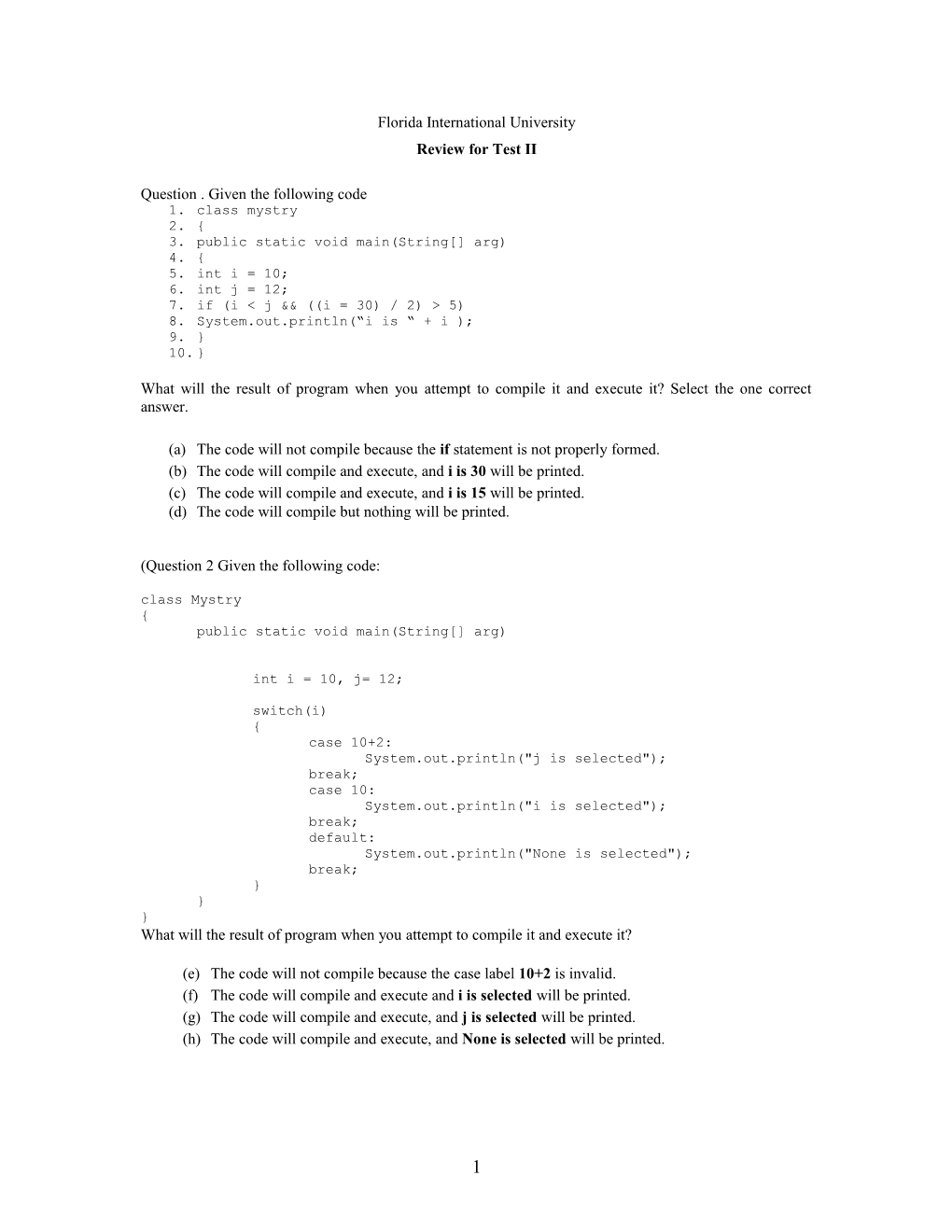# Review for Test IIFlorida International University

Review for Test II

Question . Given the following code

1. class mystry
2. {
3. public static void main(String[] arg)
4. {
5. int i = 10;
6. int j = 12;
7. if (i < j & ((i = 30) / 2) > 5)
8. System.out.println(“i is “ + i );
9. }
10. }

What will the result of program when you attempt to compile it and execute it? Select the one correct answer.

(a)The code will not compile because the if statement is not properly formed.

(b)The code will compile and execute, and i is 30 will be printed.

(c)The code will compile and execute, and i is 15 will be printed.

(d)The code will compile but nothing will be printed.

(Question 2 Given the following code:

class Mystry

{

public static void main(String[] arg)

int i = 10, j= 12;

switch(i)

{

case 10+2:

System.out.println("j is selected");

break;

case 10:

System.out.println("i is selected");

break;

default:

System.out.println("None is selected");

break;

}

}

}

What will the result of program when you attempt to compile it and execute it?

(e)The code will not compile because the case label 10+2 is invalid.

(f)The code will compile and execute and i is selected will be printed.

(g)The code will compile and execute, and j is selected will be printed.

(h)The code will compile and execute, and None is selected will be printed.

Question 3Given the following code, write down the result, as it would appear on the screen.

String place = "";

int x = -4,

y = 1;

if (x < 0)

if (y < 0)

place = place + "first";

else

place = place + "second";

place = place + "\tand third";

System.out.println(place);

Question 4Consider the following program.

1. class question
2. {
3. public static void main(String []arg)
4. {
5. int j = 2;
6. switch(j)
7. {
8. default:
9. System.out.println("value is " + j);
10. break;
11. case 2:
12. System.out.println("value is two");
13. break;
14. case 2 + 1:
15. System.out.println("value is three");
16. break;
17. }
18. }
19. }

Which of the following statement is true about the code above?

(a)The code is illegal because of the default statement is in the wrong position

(b)The whole code is illegal.

(c)Line 14 is invalid.

(d)The output would be the value is two

(e)The default statement would be executed if no option is found

Question 5 Given the following code:

1. class mystry
2. {
3. public static void main(String[] arg)
4. {
5. int i = 10;
6. int j = 12;
7. if (i < j || i == 3)
8. System.out.println(“i is “ + i );
9. }
10. }

What will the result of program when you attempt to compile it and execute it?

(i)The code will not compile because the if statement is not properly formed.

(j)The code will compile and execute and i is 3 will be printed.

(k)The code will compile and execute, and i is 10 will be printed.

(l)The code will compile but will not be executed since no object was created.

Question 6. Study the following program. Write on the line provided what will be displayed when the program is executed?

class Test

{

boolean more;

int x;

void check()

{

if (!more & x == 0)

System.out.println(x + 20);

else

System.out.println(!more);

}

}

class Driver

{

public static void main(String str[])

{

Test t = new Test();

t.check();

}

}

Question The Fast Freight Shipping Co. charges the following rate for shipping customer's package.

2 Kg or less \$1.10

Over 2 Kg but no more than 6 Kg \$2.20

6 Kg but no more than 10 Kg \$3.70

10 Kg but no more than 20 Kg \$4.80

Morethan 20 Kgcannot be shipped

• Design a class called Shipping that accepts the weight of a package and determines the charges per customer. Your class should provide methods to validate the data. This must be a separate method from the method that calculates the charges.

1